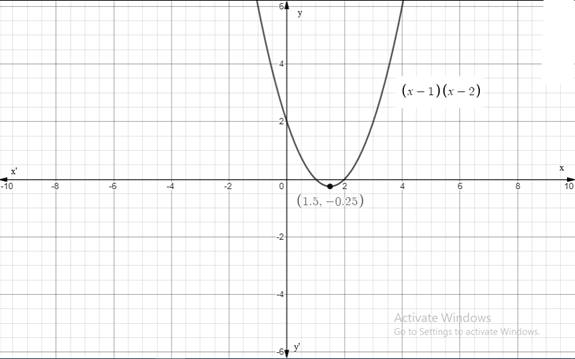# The rough graph of the parabola of the function f ( x ) = ( x − m ) ( x − n ) , x -intercepts of the graph of f , x -coordinate of the vertex of parabola in terms of m and n . Confirm the answers by expanding and with the help of formulas.### Precalculus: Mathematics for Calcu...

6th Edition
Stewart + 5 others
Publisher: Cengage Learning
ISBN: 9780840068071### Precalculus: Mathematics for Calcu...

6th Edition
Stewart + 5 others
Publisher: Cengage Learning
ISBN: 9780840068071

#### Solutions

Chapter 3.1, Problem 79E
To determine

## To find: The rough graph of the parabola of the function f(x)=(x−m)(x−n) , x -intercepts of the graph of f , x -coordinate of the vertex of parabola in terms of m and n . Confirm the answers by expanding and with the help of formulas.

Expert Solution

The graph of the parabola f(x)=(xm)(xn) is given in Figure(1) when m=1 and n=2 . The x -intercepts of the graph of f are m and n . The x -coordinate of the vertex of parabola in terms of m and n is equal to m+n2 .

### Explanation of Solution

Given information:

The function is f(x)=(xm)(xn) .

Calculation:

Take a particular case when m=1 and n=2 . Sketch the graph of the function f(x)=(xm)(xn) .Figure(1)

By investigation of the function f(x)=(xm)(xn) with the help of graphing calculator, following observations are noted:

 Function y=(x−m)(x−n) m n x -intercepts of the graph y -intercept of graph Axis of symmetry y=(x−2)(x−8) 2 8 2 and 8 16 x=5 y=(x−2)(x−3) 2 3 2 and 3 6 x=2.5 y=(x−3)(x−5) 3 5 3 and 5 15 x=4 y=(x+3)(x+5) −3 −5 −3 and −5 15 x=−4 y=(x+2)(x+4) −2 −4 −2 and −4 16 x=−3

So, from the observations it can be concluded that the x -intercepts of the graph of f are m and n .

From the table, it is visible that the x coordinate of the vertex of parabola is m+n2 .

Now check the results by expanding.

f(x)=(xm)(xn)=x2mxnx+mn=x2(m+n)x+mn

Factorize the function.

f(x)=x2(m+n)x+mn=x2(m+n)x+mn+(m+n2)2(m+n2)2=(xm+n2)2+mnm2+n2+2mn4=(xm+n2)2+4mnm2n22mn4

Further simplify,

f(x)=(xm+n2)2+4mnm2n22mn4=(xm+n2)2+m2n2+2mn4=(xm+n2)2m2+n22mn4=(xm+n2)2(mn)24

So, the x -coordinate of vertex of the parabola is m+n2 .

Now substitute f(x)=0 for the x -intercepts of parabola.

0=(xm+n2)2(mn)24(xm+n2)2=(mn)24xm+n2=±mn2x=±mn2+m+n2

Further simplify,

x=±mn2+m+n2x=mn2+m+n2,(mn)2+m+n2x=2m2,2n2x=m,n

So, the x -intercepts of parabola are m and n .

Therefore, the x -intercepts of the graph of f are m and n . The x -coordinate of the vertex of parabola in terms of m and n is equal to m+n2 .

### Have a homework question?

Subscribe to bartleby learn! Ask subject matter experts 30 homework questions each month. Plus, you’ll have access to millions of step-by-step textbook answers!